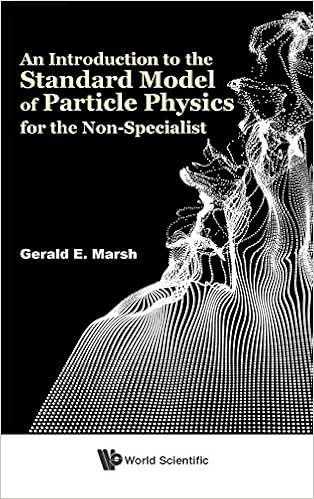# AN INTRODUCTION TO THE STANDARD MODEL OF PARTICLE PHYSICS FOR THE NON-SPECIALIST

This book takes the reader from some elementary ideas about groups to the essence of the Standard Model of particle physics along a relatively straight and intuitive path. Groups alone are first used to arrive at a classical analog  of the Dirac equation. Using elementary quantum mechanics, this analog can be turned into the actual Dirac equation, which governs the motion of the quarks and leptons of the Standard Model. After introduction to the gauge principle, the groups introduced in the beginning of the book are used to give an introduction to the Standard Model. The idea is to give an Olympian view of this evolution, one that is often missing when absorbing the detailed subject matter of the Standard Model as presented in an historical approach to the subject.